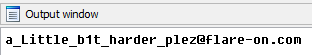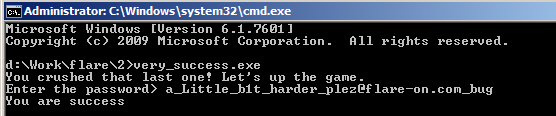# FLARE On Challenge (2015) #2

The file of challenge 2 named very_success, it is a 32 bit Windows Portable Executable file which probably written in Assembly Language. The workflow of this program is very similar to the i_am_happy_you_are_to_playing_the_flareon_challenge.exe in challenge 1. It will read a password and then verify it. Obviously, the verification algorithm is different.

In additional to the verification algorithm, the code itself looks a bit weird: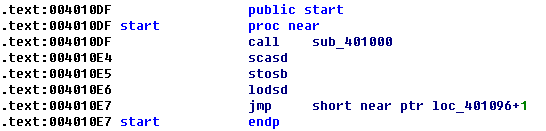There is a call at the Entry Point, followed by a piece of code which looks strange. Let’s see what will happen inside that call: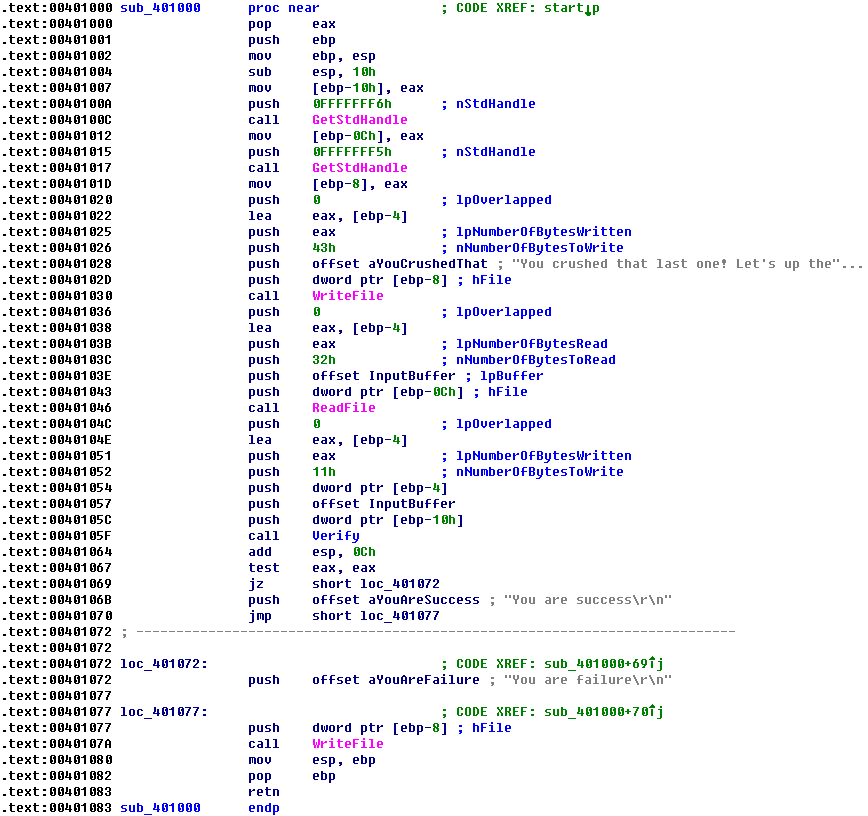If you are familiar with shellcode, you should be very sensitive about the “pop” instruction at the beginning of a call. So what is being popped out here? Yes, the return address. In this code the return address (0x004010E4) is popped to register eax, and stores to stack [ebp-10], and then it will be passed to the Verify() function located at address 0x0040105F. This explains why the code after the call at the Entry Point looks strange, it should be regarded as data instead of code!

Let’s see what will the Verify() function do: it receives three arguments, the data located at the return address (0x004010E4, I call it Key), your input (the password) and the length of the input. At the beginning of the Verify() function, it checks if the length of the input is greater than 0x25, if not it will fail. Then it verifies the input data based on the Key. The verification code here seems being “optimized” so it looks not very straightforward: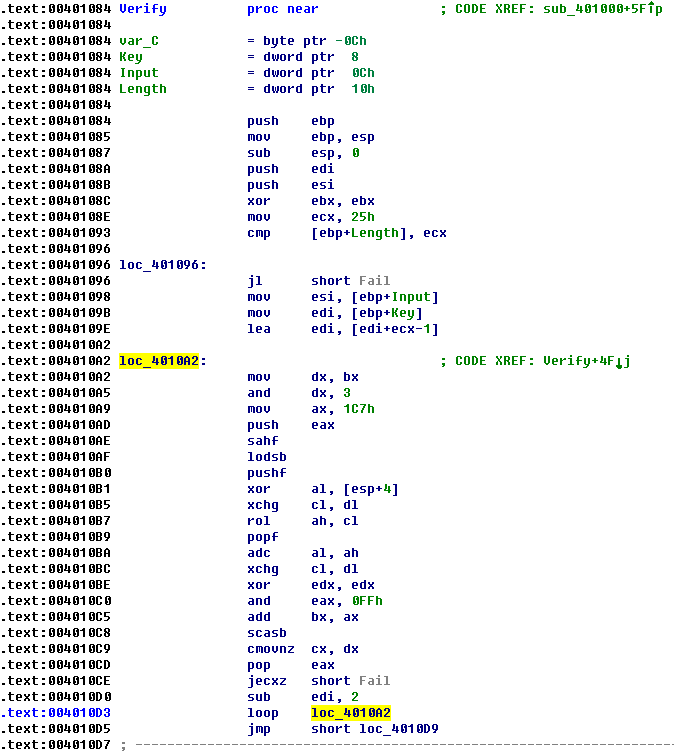Fortunately, the code is very short so there should be no difficulties for you to understand the algorithm. The following IDA python script describes the algorithm and can be used to calculate the correct password:

```from idaapi import *

def rol_byte(n, c):
if c == 0:
return n
t1 = (n << c) & 0xff
t2 = (n >> (8 - c)) & 0xff
return (t1 | t2)

length = 0x25
out = ''
c = 0

for i in range(0, length):
out += chr(((Byte(addr) - rol_byte(1, c) - 1) & 0xff) ^ 0xC7)